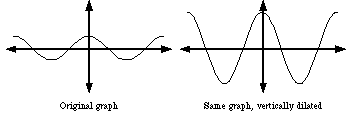dilation of a graph

Stretch
Dilation of a Graph

A transformation in which all distances on the coordinate plane are lengthened by multiplying either all x-coordinates (horizontal dilation) or all y-coordinates (vertical dilation) by a common factor greater than 1.

Note: When the common factor is less than 1 the transformation is called a compression.